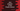# Java Program to find Simple Interest### Java program to find the Simple Interest :

In this program, we will learn how to find the simple interest using Java. To calculate simple interest, we need principal amount, rate of interest and time in year. After that , calculating the simple interest is really easy :

``````Simple Interest = (Principal * Rate of interest * time)/100
``````

### Java Program :

In this program, we will first take the inputs from the user . And then we will calculate the result and print it out.

``````import java.util.Scanner;

public class Main {

/**
* Utility functions
*/
static void println(String string) {
System.out.println(string);
}

static void print(String string) {
System.out.print(string);
}

public static void main(String[] args) {
Scanner sc = new Scanner(System.in);

println("********** Simple Interest Calculator **********");

println("Enter the amount :");
float amount = sc.nextFloat();

println("Enter Duration ( Years ):");
float year = sc.nextFloat();

println("Enter rate of Interest :");
float rate = sc.nextFloat();

float SI = (amount * year * rate) / 100;

println("Simple Interest " + SI);
}
}
``````

### Sample Output :

``````********** Simple Interest Calculator **********
Enter the amount :
10000
Enter Duration ( Years ):
3
Enter rate of Interest :
10
Simple Interest 3000.0
``````